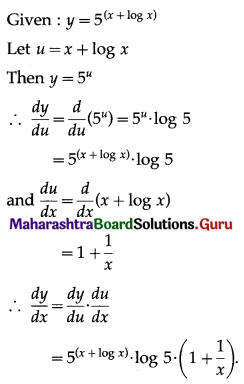# Maharashtra Board 12th Commerce Maths Solutions Chapter 3 Differentiation Ex 3.1

Balbharati Maharashtra State Board 12th Commerce Maths Solution Book Pdf Chapter 3 Differentiation Ex 3.1 Questions and Answers.

## Maharashtra State Board 12th Commerce Maths Solutions Chapter 3 Differentiation Ex 3.1

1. Find $$\frac{d y}{d x}$$ if,

Question 1.
y = $$\sqrt{x+\frac{1}{x}}$$
Solution: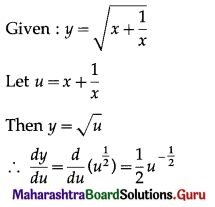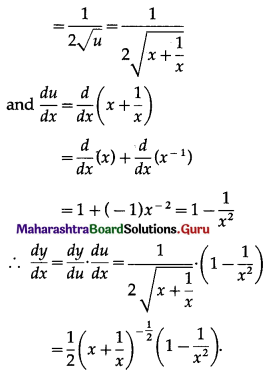Question 2.
y = $$\sqrt{a^{2}+x^{2}}$$
Solution: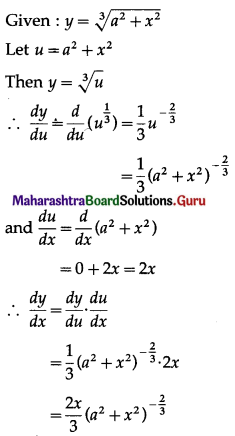Question 3.
y = (5x3 – 4x2 – 8x)9
Solution: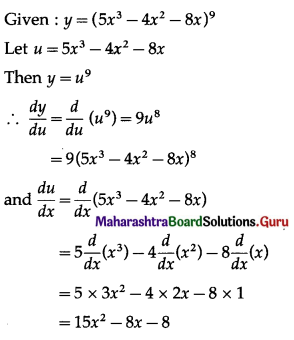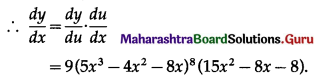2. Find $$\frac{d y}{d x}$$ if:

Question 1.
y = log(log x)
Solution:
Given y = log(log x)
Let u = log x
Then y = log u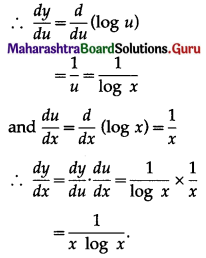Question 2.
y = log(10x4 + 5x3 – 3x2 + 2)
Solution:
Given y = log(10x4 + 5x3 – 3x2 + 2)
Let u = 10x4 + 5x3 – 3x2 + 2
Then y = log u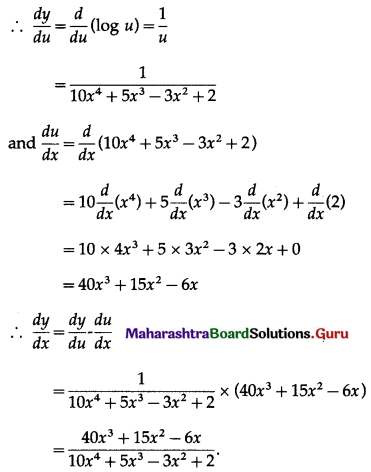Question 3.
y = log(ax2 + bx + c)
Solution:
Given y = log(ax2 + bx + c)
Let u = ax2 + bx + c
Then y = log u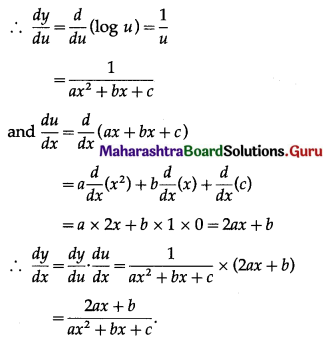3. Find $$\frac{d y}{d x}$$ if:

Question 1.
y = $$e^{5 x^{2}-2 x+4}$$
Solution: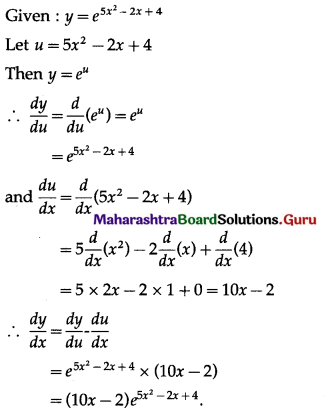Question 2.
y = $$a^{(1+\log x)}$$
Solution: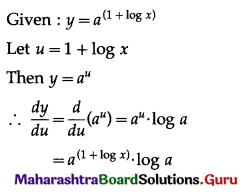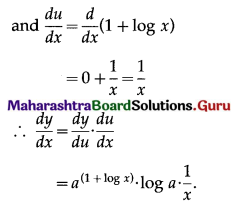Question 3.
y = $$5^{(x+\log x)}$$
Solution: